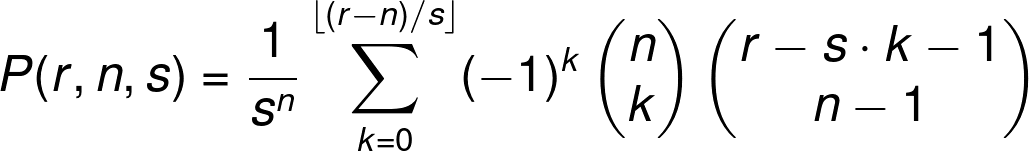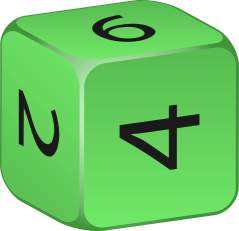# Dice Probability Calculator

Created by Wojciech Sas, PhD candidate
Reviewed by Bogna Szyk and Jack Bowater
Last updated: Mar 01, 2022

The dice probability calculator is a great tool if you want to estimate the dice roll probability over numerous variants. There are may different polyhedral die included, so you can explore the probability of a 20 sided die as well as that of a regular cubic die. So, just evaluate the odds, and play a game! In the text, you'll also find a short descriptions of each of the options.

## Polyhedral dice

Everybody knows what a regular 6 sided die is, and, most likely, many of you have already played thousands of games where the one (or more) was used. But, did you know that there are different types of die? Out of the countless possibilities, the most popular dice are included in the Dungeons & Dragons dice set, which contains seven different polyhedral dice:

• 4 sided dice, also known as a tetrahedron — each face is an equilateral triangle
• 6 sided dice, a classic cube — each face is a square
• 8 sided dice, also known as an octahedron — each face is an equilateral triangle
• 10 sided dice, also known as a pentagonal trapezohedron — each face is a kite
• 12 sided dice, also known as a dodecahedron — each face is a regular pentagon
• 20 sided dice, also known as an icosahedron — each face is an equilateral triangle

Don't worry, we take each of these dice into account in our dice probability calculator. You can choose whichever you like, and e.g. pretend to roll five 20 sided dice at once!

## How to calculate dice roll probability?

Well, the question is more complex than it seems at first glance, but you'll soon see that the answer isn't that scary! It's all about maths and statistics.

First of all, we have to determine what kind of dice roll probability we want to find. We can distinguish a few which you can find in this dice probability calculator.

Before we make any calculations, let's define some variables which are used in the formulas. n - the number of dice, s - the number of an individual die faces, p - the probability of rolling any value from a die, and P - the overall probability for the problem. There is a simple relationship - p = 1/s, so the probability of getting 7 on a 10 sided die is twice that of on a 20 sided die.

1. The probability of rolling the same value on each die - while the chance of getting a particular value on a single die is p, we only need to multiply this probability by itself as many times as the number of dice. In other words, the probability P equals p to the power n, or P = pⁿ = (1/s)ⁿ. If we consider three 20 sided dice, the chance of rolling 15 on each of them is: P = (1/20)³ = 0.000125 (or P = 1.25·10⁻⁴ in scientific notation). And if you are interested in rolling the set of any identical values, simply multiply the result by the total die faces: P = 0.000125 * 20 = 0.0025.

2. The probability of rolling all the values equal to or higher than y - the problem is similar to the previous one, but this time p is 1/s multiplied by all the possibilities which satisfy the initial condition. For example, let's say we have a regular die and y = 3. We want to rolled value to be either 6, 5, 4, or 3. The variable p is then 4 * 1/6 = 2/3, and the final probability is P = (2/3)ⁿ.

3. The probability of rolling all the values equal to or lower than y - this option is almost the same as the previous one, but this time we are interested only in numbers which are equal to or lower than our target. If we take identical conditions (s=6, y=3) and apply them in this example, we can see that the values 1, 2, & 3 satisfy the rules, and the probability is: P = (3 * 1/6)ⁿ = (1/2)ⁿ.

4. The probability of rolling exactly X same values (equal to y) out of the set - imagine you have a set of seven 12 sided dice, and you want to know the chance of getting exactly two 9s. It's somehow different than previously because only a part of the whole set has to match the conditions. This is where the binomial probability comes in handy. The binomial probability formula is:

P(X=r) = nCr * pʳ * (1-p)ⁿ⁻ʳ,

where r is the number of successes, and nCr is the number of combinations (also known as "n choose r").

In our example we have n = 7, p = 1/12, r = 2, nCr = 21, so the final result is: P(X=2) = 21 * (1/12)² * (11/12)⁵ = 0.09439, or P(X=2) = 9.439% as a percentage.

1. The probability of rolling at least X same values (equal to y) out of the set - the problem is very similar to the prior one, but this time the outcome is the sum of the probabilities for X=2,3,4,5,6,7. Moving to the numbers, we have: P = P(X=2) + P(X=3) + P(X=4) + P(X=5) + P(X=6) + P(X=7) = 0.11006 = 11.006%. As you may expect, the result is a little higher. Sometimes the precise wording of the problem will increase your chances of success.

2. The probability of rolling an exact sum r out of the set of n s-sided dice - the general formula is pretty complex:However, we can also try to evaluate this problem by hand. One approach is to find the total number of possible sums. With a pair of regular dice, we can have 2,3,4,5,6,7,8,9,10,11,12, but these results are not equivalent!

Take a look, there is only one way you can obtain 2: 1+1, but for 4 there are three different possibilities: 1+3, 2+2, 3+1, and for 12 there is, once again, only one variant: 6+6. It turns out that 7 is the most likely result with six possibilities: 1+6, 2+5, 3+4, 4+3, 5+2, 6+1. The number of permutations with repetitions in this set is 36. We can estimate the probabilities as the ratio of favorable outcomes to all possible outcomes: P(2) = 1/36, P(4) = 3/36 = 1/12, P(12) = 1/36, P(7) = 6/36 = 1/6.

🔎 Our ratio calculator can be quite helpful in finding the missing term of such ratios!

The higher the number of dice, the closer the distribution function of sums gets to the normal distribution. As you may expect, as the number of dice and faces increases, the more time is consumed evaluating the outcome on a sheet of paper. Luckily, this isn't the case for our dice probability calculator!

1. The probability of rolling a sum out of the set, not lower than X - like the previous problem, we have to find all results which match the initial condition, and divide them by the number of all possibilities. Taking into account a set of three 10 sided dice, we want to obtain a sum at least equal to 27. As we can see, we have to add all permutations for 27, 28, 29, and 30, which are 10, 6, 3, and 1 respectively. In total, there are 20 good outcomes in 1,000 possibilities, so the final probability is: P(X ≥ 27) = 20 / 1,000 = 0.02.

2. The probability of rolling a sum out of the set, not higher than X - the procedure is precisely the same as for the prior task, but we have to add only sums below or equal to the target. Having the same set of dice as above, what is the chance of rolling at most 26? If you were to do it step by step, it would take ages to obtain the result (to sum all 26 sums). But, if you think about it, we have just worked out the complementary event in the previous problem. The total probability of complementary events is exactly 1, so the probability here is: P(X ≤ 26) = 1 - 0.02 = 0.98.

## When to use dice probability calculator?

There are a lot of board games where you take turns to roll a die (or dice), and the results may be used in numerous contexts. Let's say you're playing Dungeons & Dragons and attacking. Your opponent's armor class is 17. You roll a 20 sided dice, hoping for a result of at least 15 - with your modifier of +2, that should be enough. With these conditions, the probability of a successful attack is 0.30. If you know the odds of a successful attack, you can choose whether you want to attack this target or pick another with better odds.

Or maybe you're playing The Settlers of Catan and you hope to roll the sum of exactly 8 with two 6 sided dice, as this result will yield you precious resources. Just use our dice probability calculator, and you'll see the chance is around 0.14 - you'd better get lucky this turn!

## Should I play or should I pass? - Let's play a game!

There are various types of game, like lotteries, where your task is to make a bet depending on the odds. Rolling dice is one of them. Although it's inevitable to take some risk, you can choose the most favorable option, and maximize your chances of a win. Take a look at this example.

Imagine you are playing a game where you have one of three options to choose from, which are:

• the sum of five 10 sided dice is at least 30
• the sum of five 12 sided dice is at most 28
• the sum of five 20 sided dice is at least 59

You only win if the option you pick comes up. You can also pass if you feel none of these will happen. Intuitively, it's difficult to estimate the most likely success, but with our dice probability calculator, it takes only a blink of an eye to evaluate all the probabilities.

The resulting values are:

• P₁ = 0.38125 for 10 sided dice
• P₂ = 0.3072 for 12 sided dice
• P₃ = 0.3256 for 20 sided dice.

The probability for a pass to be successful is the product of the complementary events of the remaining options:

• P₄ = (1-P₁) * (1-P₂) * (1-P₃) = 0.61875 * 0.6928 * 0.6744 = 0.2891.

We can see that the most favorable option is the first one, while passing is the least likely event to happen. We cannot assure you'll win all the time, but we strongly recommend that you pick the 10 sided dice set to play.

Wojciech Sas, PhD candidate
Choose the type and the number of dice
Dice type
Cube (6 faces)Total number of dice
Choose the conditions
Game rules
All dice with the same value
The value
...which results in...
The probability (for any value)
People also viewed…

### Black Hole Collision Calculator

The Black Hole Collision Calculator lets you see the effects of a black hole collision, as well as revealing some of the mysteries of black holes, come on in and enjoy!

### Confidence interval

The confidence interval calculator finds the confidence level for your data sample.

### Sleep

The sleep calculator can help you determine when you should go to bed to wake up happy and refreshed.

### Upper control limit

The upper control limit calculator helps you determine the lower and upper control limit values of your dataset.